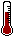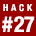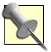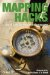# Hack 27. Calculate the Distance Between Points on the Earths Surface

### Hack 27 Calculate the Distance Between Points on the Earth s SurfaceA little spherical trigonometry can go a long way.

The task of calculating the distance between two points on the Earth's surface is not quite as simple as it might seem. At first, we might be inclined to dust off the well-known Pythagorean Theorem from high school algebra, which calculates the length of the hypotenuse of a right triangle.

`a2 + b2 = c2`

If we have two points (x1, y1) and (x2, y2), then the difference between our two x-coordinates and the difference between our two y-coordinates are the legs of our right triangle, and the hypotenuse measures the distance between the points:

```c = sqrt
( (x2 - x1)2 + (y2 - y1)2 )```

One hitch to this approach is that the distance between lines of longitude decreases as you head toward the poles, which is a symptom of the fact that, in contrast to our right triangle, the Earth isn't flat. (Damn you, Columbus!) Worse, over distances greater than 20 kilometers or so, the curvature of the Earth turns our straight hypotenuse into an arc, making the Pythagorean Theorem more or less useless for this purpose. This arc, if we were to extend it all the way around the world through both points, describes a figure known as a great circle. The distance between two points on the Earth is therefore sometimes referred to as the great-circle distance. We show how to draw great circles in [Hack #30] .You can still use the Pythagorean Theorem for calculating distances reliably in rectangular coordinate systems like UTM, which is one compelling reason to use these systems instead of latitude and longitude, as discussed in [Hack #26] .

In order to determine the great-circle distance between two points, we can apply the Law of Cosines, which is itself a generalization of the Pythagorean theorem to triangles that lack a right angle. The Law of Cosines for spherical trigonometry states that:

`cos c = cos a cos b + sin a sin b cos C`

In this relation, C is the angle between edges a and b on a "triangle" connecting three points on a spherical surface. If we place the third vertex of our spherical triangle at the North Pole, then the edges a and b, which connect our two points to the third vertex, can be measured in units of latitude, and angle C conveniently becomes the difference in longitude between them. Figure 3-15 depicts this relationship, using the great circle between Lagos, Nigeria, and San Francisco, California. We can then apply the inverse cosine function to the Law of Cosines to get the length of edge c, which is the distance between our two original points:

```c = cos-1( cos(90 - lat1) cos(90 - lat2)
+ sin(90 - lat1) sin(90 - lat2) cos(lon2 - lon1) )```

Figure 3-15. The Law of Cosines, exemplified with the great circle connecting San Francisco and Lagos3.7.1. The Code

However, our work is not quite done yet. For starters, most computational implementations of trigonometric functions take arguments measured in radians, not degrees. (Degrees can be converted to radians by multiplying by / 180.) Also, the result c is returned in radians (i.e., a proportion of the radius of a unit sphere), which needs to be scaled to a real world measure to be useful. The following Perl code incorporates these extra steps:

```use Math::Trig;

use constant RADIUS => 6367; # kilometers

sub great_circle {
my (\$lat1, \$lon1, \$lat2, \$lon2) = @_;

my \$a = deg2rad( 90 - \$lat1 );
my \$b = deg2rad( 90 - \$lat2 );
my \$theta = deg2rad( \$lon2 - \$lon1 );
my \$c = acos( cos(\$a) * cos(\$b) +
sin(\$a) * sin(\$b) * cos(\$theta) );

}```

The Math::Trig module, which comes with Perl, supplies the deg2rad() and acos( ) functions. Math::Trig also supplies a great_circle_distance() function, which can be used to simplify the preceding code as follows:

```use Math::Trig 'great_circle_distance';

use constant RADIUS => 6367; # kilometers

sub great_circle {
my (\$lat1, \$lon1, \$lat2, \$lon2) = map(deg2rad(\$_), @_);
return great_circle_distance( \$lon1, \$lat1, \$lon2, \$lat2, RADIUS );
}```As usual, latitudes south of the equator and longitudes west of the prime meridian should be given as negative values. Also, as is common with GIS-related tools, great_circle_distance() takes its arguments with longitude first, because, after all, longitude is the x-coordinate and latitude the y- coordinate.

The functionality embodied in our great_circle() function is also provided by the Geo::Distance module, which can be downloaded from the CPAN. Geo::Distance provides a nice wrapper for this method, allowing us to specify our units in English:

``` use Geo::Distance 'distance_calc';

my \$dist_in_kilometers =
distance_calc( kilometer => \$lon1, \$lat1, \$lon2, \$lat2 );

my \$dist_in_miles =
distance_calc( mile => \$lon1, \$lat1, \$lon2, \$lat2 );```

Geo::Distance has a few other nice features; see perldoc Geo::Distance for more details.

3.7.2. Other Considerations

Conventional wisdom holds that this approach has one major drawback, in that it relies on the mathematical properties of the inverse cosine function, which suffers badly from rounding errors for distances separated by less than a few degrees of arc. As an alternative, the formula given by the Law of Cosines can be rewritten using various trigonometric identities to yield the Haversine formula, which does not rely on the inverse cosine function to calculate great-circle distances.

However, with the number of significant figures used in modern double-precision floating math, the rounding errors expected with the inverse cosine function are actually not typically an issue. In fact, in temperate latitudes, the inverse-cosine formula for great-circle distances appears to be substantially more accurate than the Haversine formula. For distances wholly within the polar latitudes, the Haversine formula may or may not be more accurate.

The attentive reader may have noticed one other potentially serious hitch, which we have heretofore glossed over: although Earth is most certainly not flat, it is not exactly round, either. Rather, Earth is an oblate spheroid, slightly flattened at the poles. The WGS84 geodetic datum gives Earth an equatorial (or semimajor) radius of 6,378 kilometers, but a polar (or semiminor) radius of only 6,356 kilometersa difference of about 22 kilometers. The value we used earlier is simply the arithmetic mean of these two values. Does the difference matter? For sake of argument, let's calculate the distance from London (51.52N, 0.10W) to Sydney (33.87S, 151.21E) using the two extremes:

```use Geo::Distance ':all';

reg_unit( 'semimajor', 6378 );
reg_unit( 'semiminor', 6356 );

print "\$_: ", distance_calc( \$_, -0.10, 51.52, 151.21, -33.87 ), "
"
for qw( semimajor semiminor );```

We use the reg_unit() function to register our own units for the radius of Earth and then call distance_calc() with each of our custom units in order to compare them. The result looks like this:

```semimajor: 17010.3827750906
semiminor: 16951.7078893816```

The difference between using the semimajor and semiminor radii of Earth yields a discrepancy of almost 60 kilometers! Looked at one way, this distance equals half a degree of longitude, but, looked at another way, it's only a 0.35% difference in measuring a line that stretches halfway across the world. Using the mean of the semimajor and semiminor radii mitigates the potential for error somewhat, but, obviously, the amount of time you spend considering this issue should bear some relation to the actual degree of accuracy you're after.

• [Hack #30]
• [Hack #26]
• Sinnott, R.W. "Virtues of the Haversine." Sky and Telescope, Vol. 68, No. 2, 1984, p. 159.
• See http://mappinghacks.com/projects/distance/ for a discussion of different ways to simplify distance calculations.Mapping Hacks: Tips & Tools for Electronic Cartography
ISBN: 0596007035
EAN: 2147483647
Year: 2004
Pages: 172

Similar book on Amazon